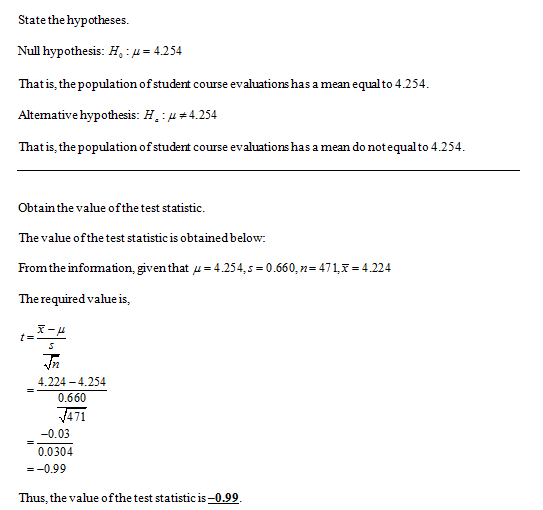# A data set includes data from student evaluations of courses at a particular university. The summary statistics aren equals=471,x overbarx equals=4.224,sequals=0.660Use a 0.050 significance level to test the claim that the population of student course evaluations has a mean equal to 4.254. Assume that a simple random sample has been selected. Do the results apply to the population of all​ students?

Question
12 views

A data set includes data from student evaluations of courses at a particular university. The summary statistics are

n equals=471,
x overbarx equals=4.224,
sequals=0.660
Use a 0.050 significance level to test the claim that the population of student course evaluations has a mean equal to 4.254. Assume that a simple random sample has been selected. Do the results apply to the population of all​ students?
check_circle

Step 1...

### Want to see the full answer?

See Solution

#### Want to see this answer and more?

Solutions are written by subject experts who are available 24/7. Questions are typically answered within 1 hour.*

See Solution
*Response times may vary by subject and question.
Tagged in